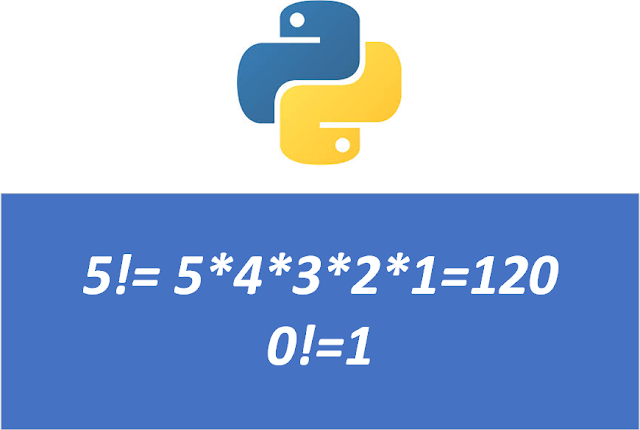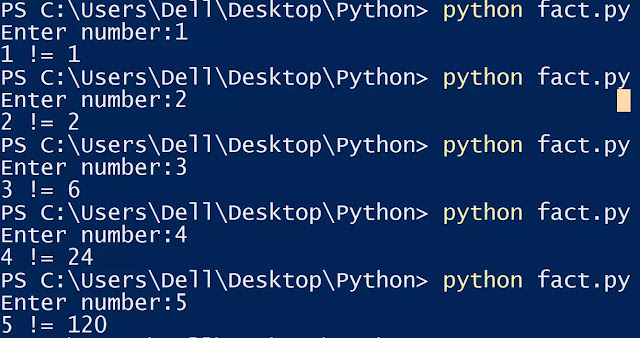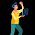# Python Program to Find Factorial of a Number using for loop

In this post, I have provided coding and a quick algorithm to make a Python program to find factorial of a number and Factorial of a number in python using recursion.
I have a 5-minute task for you today. If you do it, it will clear all your doubts regarding the Python program to find the factorial of a number.

Factorial = It is the product of all positive integers less than or equal to that number.
It is denoted by “!”• Now, we have to make a python program that takes the number from the user and it has to calculate the factorial of that number. It will simply print factorial of that number. The factorial of that number is calculated using for loop.

## Python program to find factorial of a number using for loop

```n=int(input("Enter number:"))
fact=1
for i in range(1,n+1,1):
fact=fact*i
print(n,"!=",fact)
```

Output:Enter number:8
8 != 40320.

This program obtains an integer input from the user. Then using for loop, we will calculate the factorial of that number.

## Quick Algo for factorial Program in Python using for loop:

1. Input an integer number from the user.
2. Initialize fact=1.
3. Use for loop, to multiply fact with all the numbers less than and equal to the number given by the user.
4. Now, simply print the factorial of that number.If you need the source code of any other program, write in the comment section.
Your 5-minute task for today is to write a Factorial Program in Python using recursion. In recursion, the factorial function calls itself until it reaches factorial 1.

## Factorial of a number in python using recursion

In this post, we are going to make a factorial program in python using a recursive function.
Recursive function are those functions which call the same function within the body

```def factorial(n):
if n==1:
return 1
else:
return n*factorial(n-1)
n=int(input("Enter number:"))
print('Factorial:',factorial(n))
```

Output:

Enter number:6
Factorial: 720

This is all about the Python program to find the factorial of a number. If you are having any issues or doubts related to this program, let me know in the comment section.

.
Related posts:• Hackerrank 5* • Full Stack Developer • Masters in SEO

### Related Posts

1.2.